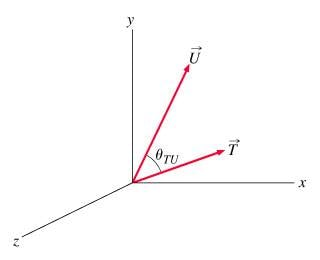Cross product homework

1. Find the sine of the angle between and .2. AxB = ABsin(alpha)

3. I know T = (3,1,0) and U = (2,4,0), and I calculated that V = TxU = (0,0,10), so the magnitude of V is 10.

Then I did 10 = sqrt(10)*sqrt(20)*sin(alpha)

Divided 10 by sqrt(10)*sqrt(20), took the inverse sine, and obtained 45 degrees, which is incorrect.

Mentallic
Homework Helper
I haven't studied 3-D vectors, but this problem can be simplified into a 2-D diagram, and quite easily since z=0 for both vectors.
I also got the answer of 45o by answering it with trigonometry.

But the question does say find the sine of the angle, correct? So are we looking for the answer $1/\sqrt{2}$ ?

We are not finding sine(theta), we are finding theta, which is what I calculated.

HallsofIvy
You have already been told that 45 degrees ($\pi/4$ radians) is the correct answer. What makes you say it is incorrect?
As a check, use the fact that $\vec{u}\cdot\vec{v}= |\vec{u}||\vec{v}|cos(\theta)$. Since, here, $|\vec{u}\times\vec{v}|= \vec{u}\cdot\vec{v}$, $sin(\theta)= cos(\theta)$ and the angle, being in the first quadrant must be $\pi/4$.